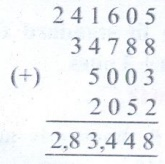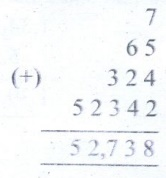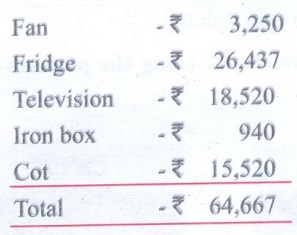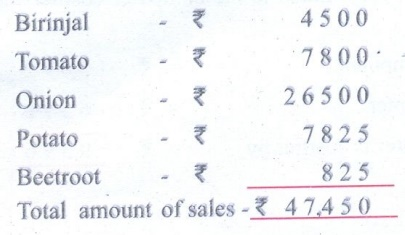Home | | Maths 5th Std | Exercise 2.5 (Addition)

## Chapter: 5th Maths : Term 1 Unit 2 : Numbers

Text Book Back Exercises Questions with Answers, Solution : 5th Maths : Term 1 Unit 2 : Numbers : Exercise 2.5 (Addition)

Exercise 2.5

1. Find the sum.a. 19732 + 24105 + 525 + 48b. 241605 + 34788 + 5003 + 2052c. 1000 + 250787 + 3574 + 43d. 7 + 65 + 324 + 52342.3. population of 5 villages in a town panchayat are 980, 3254, 4125, 687and 6786. What is the total population?4. Ramu bought some home needs. The price list of which is given below. What is the total cost ?5. A day sales of different vegetables in a vegetable shop is as follows: Brinjals is ₹ 4500, Tomato is ₹ 7800, Onion is ₹ 26,500, Potato is ₹ 7825 and Beetroot is ₹ 825. Fund the total amount of sales?Exercise 2.5

1. 61,866; 41,969

2. a) 44,410 b) 2,83, 448 c) 2, 55, 404 d) 52, 738

3. 15,832

4. Rs. 64, 667

5. Rs. 47,450

Tags : Numbers | Term 1 Chapter 2 | 5th Maths , 5th Maths : Term 1 Unit 2 : Numbers
Study Material, Lecturing Notes, Assignment, Reference, Wiki description explanation, brief detail
5th Maths : Term 1 Unit 2 : Numbers : Exercise 2.5 (Addition) | Numbers | Term 1 Chapter 2 | 5th Maths